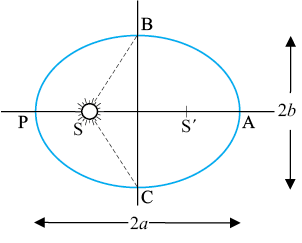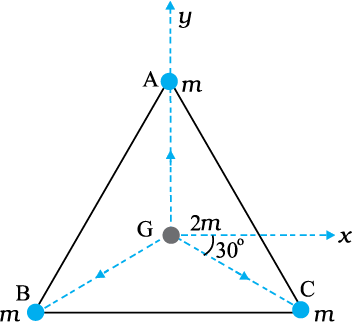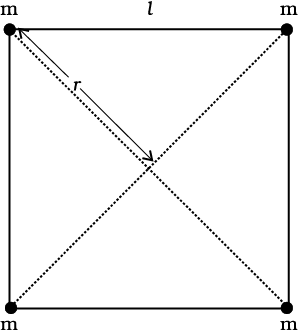Let the speed of the planet at the perihelion in figure shown below be ${\mathrm{v}}_{\mathrm{P}}$ and the Sun-planet distance SP be ${\mathrm{r}}_{\mathrm{P}}$. Relation between  to the corresponding quantities at the aphelion  is:1.

2.

3.

4.   None of these

Subtopic:  Kepler's Laws |
To view explanation, please take trial in the course below.
NEET 2023 - Target Batch - Aryan Raj Singh
To view explanation, please take trial in the course below.
NEET 2023 - Target Batch - Aryan Raj Singh
Launched MCQ Practice Books

Prefer Books for Question Practice? Get NEETprep's Unique MCQ Books with Online Audio/Video/Text Solutions via Telegram Bot

Let the speed of the planet at the perihelion in figure shown below be ${\mathrm{v}}_{\mathrm{P}}$ and the Sun-planet distance SP be ${\mathrm{r}}_{\mathrm{P}}$. Will the planet take equal times to traverse BAC and CPB ?1.   No

2.   Yes

3.   Depends on mass of planet

4.   We can't say anything

Subtopic:  Kepler's Laws |
To view explanation, please take trial in the course below.
NEET 2023 - Target Batch - Aryan Raj Singh
To view explanation, please take trial in the course below.
NEET 2023 - Target Batch - Aryan Raj Singh
Launched MCQ Practice Books

Prefer Books for Question Practice? Get NEETprep's Unique MCQ Books with Online Audio/Video/Text Solutions via Telegram Bot

Three equal masses of m kg each are fixed at the vertices of an equilateral triangle ABC. What is the force acting on a mass 2m placed at the centroid G of the triangleTake AG = BG = CG = 1 m.1.   ${\mathrm{Gm}}^{2}\left(\stackrel{^}{\mathrm{i}}+\stackrel{^}{\mathrm{j}}\right)$

2.   ${\mathrm{Gm}}^{2}\left(\stackrel{^}{\mathrm{i}}-\stackrel{^}{\mathrm{j}}\right)$

3.   0

4.   $2{\mathrm{Gm}}^{2}\left(\stackrel{^}{\mathrm{i}}+\stackrel{^}{\mathrm{j}}\right)$

Subtopic:  Newton's Law of Gravitation |
To view explanation, please take trial in the course below.
NEET 2023 - Target Batch - Aryan Raj Singh
To view explanation, please take trial in the course below.
NEET 2023 - Target Batch - Aryan Raj Singh
Launched MCQ Practice Books

Prefer Books for Question Practice? Get NEETprep's Unique MCQ Books with Online Audio/Video/Text Solutions via Telegram Bot

Three equal masses of m kg each are fixed at the vertices of an equilateral triangle ABC. What is the force acting on a mass 2m placed at the centroid G of the triangle if the mass at the vertex A is doubled? Take AG = BG = CG = 1 m.1.

2.

3.   0

4.

Subtopic:  Newton's Law of Gravitation |
To view explanation, please take trial in the course below.
NEET 2023 - Target Batch - Aryan Raj Singh
To view explanation, please take trial in the course below.
NEET 2023 - Target Batch - Aryan Raj Singh
Launched MCQ Practice Books

Prefer Books for Question Practice? Get NEETprep's Unique MCQ Books with Online Audio/Video/Text Solutions via Telegram Bot

The potential energy of a system of four particles placed at the vertices of a square of side l (as shown in the figure below) and the potential at the centre of the square, respectively, are:1.   $-5.41\frac{{\mathrm{Gm}}^{2}}{\mathrm{l}}$ and 0

2.   0 and $-5.41\frac{{\mathrm{Gm}}^{2}}{\mathrm{l}}$

3.   $-5.41\frac{{\mathrm{Gm}}^{2}}{\mathrm{l}}$ and $-4\sqrt{2}\frac{\mathrm{Gm}}{\mathrm{l}}$

4.   0 and 0

Subtopic:  Gravitational Potential Energy |
To view explanation, please take trial in the course below.
NEET 2023 - Target Batch - Aryan Raj Singh
To view explanation, please take trial in the course below.
NEET 2023 - Target Batch - Aryan Raj Singh
Launched MCQ Practice Books

Prefer Books for Question Practice? Get NEETprep's Unique MCQ Books with Online Audio/Video/Text Solutions via Telegram Bot

Two uniform solid spheres of equal radii R, but mass and 4have a centre to centre separation 6R, as shown in the figure. The two spheres are held fixed. A projectile of mass is projected from the surface of the sphere of mass directly towards the centre of the second sphere. The expression for the minimum speed v of the projectile so that it reaches the surface of the second sphere is:1.   ${\left(\frac{3\mathrm{GM}}{5\mathrm{R}}\right)}^{1/2}$

2.   ${\left(\frac{2\mathrm{GM}}{5\mathrm{R}}\right)}^{1/2}$

3.   ${\left(\frac{3\mathrm{GM}}{2\mathrm{R}}\right)}^{1/2}$

4.   ${\left(\frac{5\mathrm{GM}}{3\mathrm{R}}\right)}^{1/2}$

Subtopic:  Gravitational Potential Energy |
To view explanation, please take trial in the course below.
NEET 2023 - Target Batch - Aryan Raj Singh
To view explanation, please take trial in the course below.
NEET 2023 - Target Batch - Aryan Raj Singh
Launched MCQ Practice Books

Prefer Books for Question Practice? Get NEETprep's Unique MCQ Books with Online Audio/Video/Text Solutions via Telegram Bot

The planet Mars has two moons, Phobos and Delmos. Phobos has a period of 7 hours, 39 minutes and an orbital radius of $9.4×{10}^{3}$ km. The mass of mars is:

1.

2.

3.

4.

Subtopic:  Satellite |
To view explanation, please take trial in the course below.
NEET 2023 - Target Batch - Aryan Raj Singh
To view explanation, please take trial in the course below.
NEET 2023 - Target Batch - Aryan Raj Singh
Launched MCQ Practice Books

Prefer Books for Question Practice? Get NEETprep's Unique MCQ Books with Online Audio/Video/Text Solutions via Telegram Bot

You are given the following data: g 9.81 $\mathrm{m}/{\mathrm{s}}^{2}$,  m, the distance to the moon, R = $3.84×{10}^{8}$ m and the time period of the moon’s revolution is 27.3 days. Mass of the Earth ${\mathrm{M}}_{\mathrm{E}}$ in two different ways is:

1.

2.

3.

4.

Subtopic:  Satellite |
To view explanation, please take trial in the course below.
NEET 2023 - Target Batch - Aryan Raj Singh
To view explanation, please take trial in the course below.
NEET 2023 - Target Batch - Aryan Raj Singh
Launched MCQ Practice Books

Prefer Books for Question Practice? Get NEETprep's Unique MCQ Books with Online Audio/Video/Text Solutions via Telegram Bot

Constant in days and kilometres is?

1.

2.

3.

4.

Subtopic:  Satellite |
To view explanation, please take trial in the course below.
NEET 2023 - Target Batch - Aryan Raj Singh
To view explanation, please take trial in the course below.
NEET 2023 - Target Batch - Aryan Raj Singh
Launched MCQ Practice Books

Prefer Books for Question Practice? Get NEETprep's Unique MCQ Books with Online Audio/Video/Text Solutions via Telegram Bot

The moon is at a distance of $3.84×{10}^{5}$ km from the earth. Its time period of revolution in days is: (Given )

1.   17.3 days

2.   33.7 days

3.   27.3 days

4.   4 days

Subtopic:  Satellite |
To view explanation, please take trial in the course below.
NEET 2023 - Target Batch - Aryan Raj Singh
To view explanation, please take trial in the course below.
NEET 2023 - Target Batch - Aryan Raj Singh